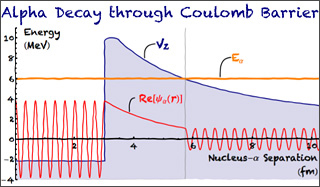22.02 | Spring 2012 | Undergraduate
Introduction to Applied Nuclear Physics

## 22-02s12.jpg

Description:

This graph depicts the Gamow model of alpha decay, in which an alpha particle tunnels through a Coulomb potential barrier. Figure by Prof. Paola Cappellaro, inspired by a Wolfram blog post about creating xkcd-style Mathematica plots.

Alt text:
Graph showing the alpha particle wavefunction passing through a large nuclear potential barrier.
Caption:
This graph depicts the Gamow model of alpha decay, in which an alpha particle tunnels through a Coulomb potential barrier. (Figure by Prof. Paola Cappellaro, inspired by a Wolfram blog post about creating xkcd-style Mathematica plots.)
Credit:
Figure by Prof. Paola Cappellaro, inspired by a Wolfram blog post about creating xkcd-style Mathematica plots.